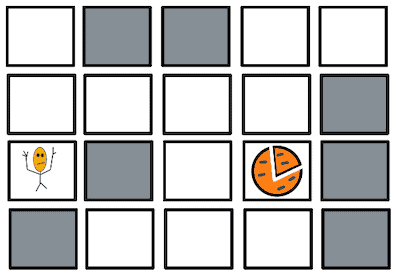# Shortest Path to Get FoodLeetcode 1730. Shortest Path to Get Food

##### Problem

You are starving and you want to eat food as quickly as possible. You want to find the shortest path to arrive at any food cell.

You are given an `m x n` character matrix, `grid`, of these different types of cells:

• `'*'` is your location. There is exactly one `'*'` cell.
• `'#'` is a food cell. There may be multiple food cells.
• `'O'` is free space, and you can travel through these cells.
• `'X'` is an obstacle, and you cannot travel through these cells.

You can travel to any adjacent cell north, east, south, or west of your current location if there is not an obstacle.

Return the length of the shortest path for you to reach any food cell. If there is no path for you to reach food, return `-1`.

Example 1:

```Input: grid = [["X","X","X","X","X","X"],["X","*","O","O","O","X"],["X","O","O","#","O","X"],["X","X","X","X","X","X"]]
Output: 3
Explanation: It takes 3 steps to reach the food.
```

Example 2:

```Input: grid = [["X","X","X","X","X"],["X","*","X","O","X"],["X","O","X","#","X"],["X","X","X","X","X"]]
Output: -1
Explanation: It is not possible to reach the food.
```

Example 3:

```Input: grid = [["X","X","X","X","X","X","X","X"],["X","*","O","X","O","#","O","X"],["X","O","O","X","O","O","X","X"],["X","O","O","O","O","#","O","X"],["X","X","X","X","X","X","X","X"]]
Output: 6
Explanation: There can be multiple food cells. It only takes 6 steps to reach the bottom food.```

Constraints:

• `m == grid.length`
• `n == grid[i].length`
• `1 <= m, n <= 200`
• `grid[row][col]` is `'*'``'X'``'O'`, or `'#'`.
• The `grid` contains exactly one `'*'`.
##### Solution

This problem can be solved by a straight forward implementation of Breadth First Search algorithm. If we were asked to take all the foods with minimum length, the story would have been different though.

To apply BFS, we can take a queue and push the coordinates of source. Until we reach the coordinate of a single food, we will continue exploring neighbour nodes and push into the queue.

During this process, we will also keep track of distance passed so far. Once one of the food coordinates is found, we will return the destination so far plus one.

Below code is pretty simple to understand the above algorithm.

###### C++ Code
```class Solution {
public:
int getFood(vector<vector<char>>& grid) {

queue<pair<int, int>> q;
int R = grid.size();
if(R == 0)
return -1;

int C = grid.size();
if(C == 0)
return -1;

vector<vector<int>> visited(R, vector<int>(C, 0));
for(int i = 0; i < R; i++){
for(int j = 0; j < C; j++){
if(grid[i][j] == '*'){
q.push({i, j});
visited[i][j] = 1;
break;
}
}
}
vector<vector<int>> dir{ {-1, 0}, {1, 0}, {0, -1}, {0, 1} };
int distance = 0;
while(!q.empty()){
int len = q.size();
for(int l = 0; l < len; l++){
pair<int, int> pr = q.front();
q.pop();
for(int i = 0; i < 4; i++){
int r = pr.first + dir[i];
int c = pr.second + dir[i];
if(0 <= r && r < R && 0 <= c && c < C){
if(grid[r][c] == '#')
return distance + 1;
if(grid[r][c] == 'O' && visited[r][c] == 0){
q.push({r, c});
visited[r][c] = 1;
}
}
}
}
distance += 1;
}
return -1;
}
};```